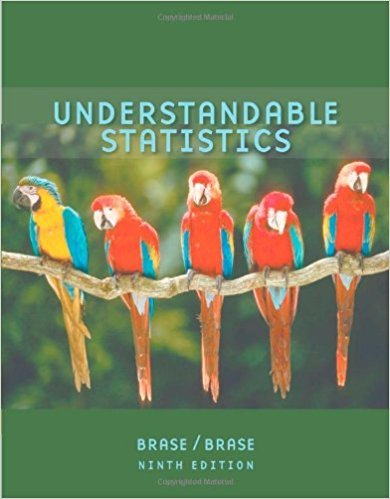×
×

# Solutions for Chapter 5.2: The Binomial Probability Distribution and Related Topics## Full solutions for Understandable Statistics | 9th Edition

ISBN: 9780618949922Solutions for Chapter 5.2: The Binomial Probability Distribution and Related Topics

Solutions for Chapter 5.2
4 5 0 349 Reviews
25
0
##### ISBN: 9780618949922

This expansive textbook survival guide covers the following chapters and their solutions. Chapter 5.2: The Binomial Probability Distribution and Related Topics includes 24 full step-by-step solutions. Understandable Statistics was written by and is associated to the ISBN: 9780618949922. Since 24 problems in chapter 5.2: The Binomial Probability Distribution and Related Topics have been answered, more than 35772 students have viewed full step-by-step solutions from this chapter. This textbook survival guide was created for the textbook: Understandable Statistics, edition: 9.

Key Statistics Terms and definitions covered in this textbook

A variation of the R 2 statistic that compensates for the number of parameters in a regression model. Essentially, the adjustment is a penalty for increasing the number of parameters in the model. Alias. In a fractional factorial experiment when certain factor effects cannot be estimated uniquely, they are said to be aliased.

• Alias

In a fractional factorial experiment when certain factor effects cannot be estimated uniquely, they are said to be aliased.

• Alternative hypothesis

In statistical hypothesis testing, this is a hypothesis other than the one that is being tested. The alternative hypothesis contains feasible conditions, whereas the null hypothesis speciies conditions that are under test

• Analytic study

A study in which a sample from a population is used to make inference to a future population. Stability needs to be assumed. See Enumerative study

• Average

See Arithmetic mean.

• Consistent estimator

An estimator that converges in probability to the true value of the estimated parameter as the sample size increases.

• Contour plot

A two-dimensional graphic used for a bivariate probability density function that displays curves for which the probability density function is constant.

• Control chart

A graphical display used to monitor a process. It usually consists of a horizontal center line corresponding to the in-control value of the parameter that is being monitored and lower and upper control limits. The control limits are determined by statistical criteria and are not arbitrary, nor are they related to speciication limits. If sample points fall within the control limits, the process is said to be in-control, or free from assignable causes. Points beyond the control limits indicate an out-of-control process; that is, assignable causes are likely present. This signals the need to ind and remove the assignable causes.

• Correlation

In the most general usage, a measure of the interdependence among data. The concept may include more than two variables. The term is most commonly used in a narrow sense to express the relationship between quantitative variables or ranks.

• Critical region

In hypothesis testing, this is the portion of the sample space of a test statistic that will lead to rejection of the null hypothesis.

• Cumulative distribution function

For a random variable X, the function of X deined as PX x ( ) ? that is used to specify the probability distribution.

• Defect concentration diagram

A quality tool that graphically shows the location of defects on a part or in a process.

• Density function

Another name for a probability density function

• Design matrix

A matrix that provides the tests that are to be conducted in an experiment.

• Fractional factorial experiment

A type of factorial experiment in which not all possible treatment combinations are run. This is usually done to reduce the size of an experiment with several factors.

• Gamma function

A function used in the probability density function of a gamma random variable that can be considered to extend factorials

• Gaussian distribution

Another name for the normal distribution, based on the strong connection of Karl F. Gauss to the normal distribution; often used in physics and electrical engineering applications

• Generating function

A function that is used to determine properties of the probability distribution of a random variable. See Moment-generating function

• Geometric mean.

The geometric mean of a set of n positive data values is the nth root of the product of the data values; that is, g x i n i n = ( ) = / w 1 1 .

• Geometric random variable

A discrete random variable that is the number of Bernoulli trials until a success occurs.

×